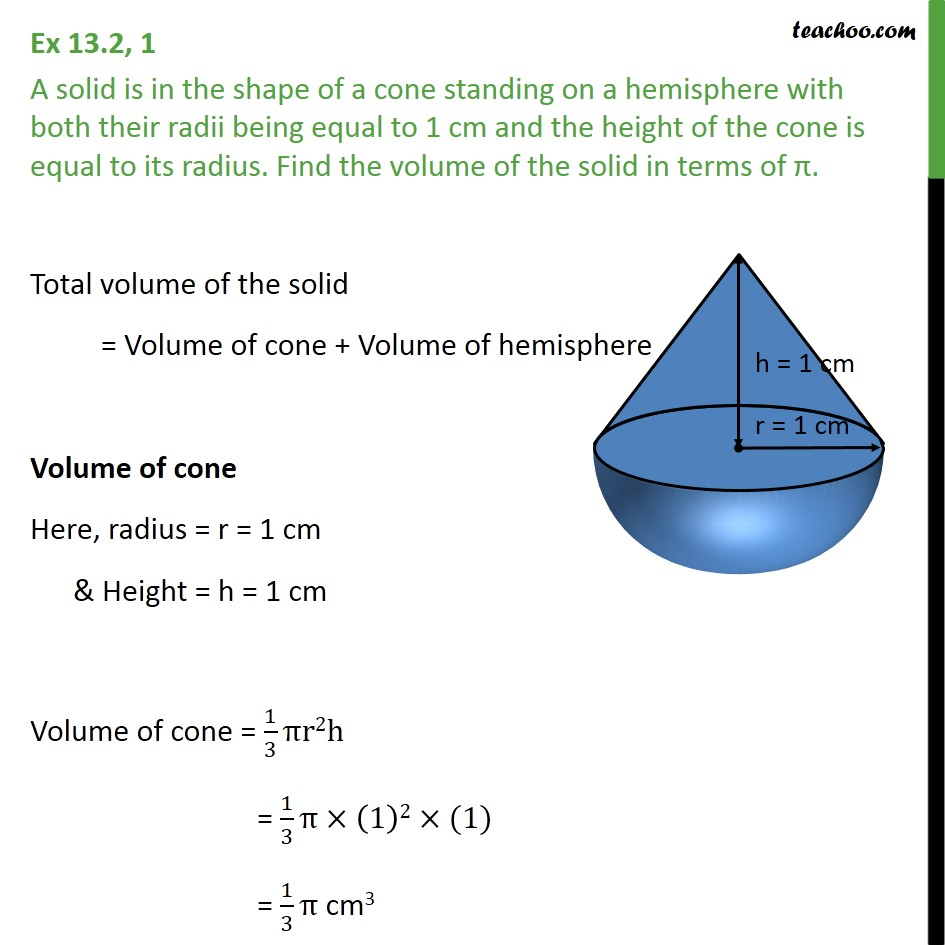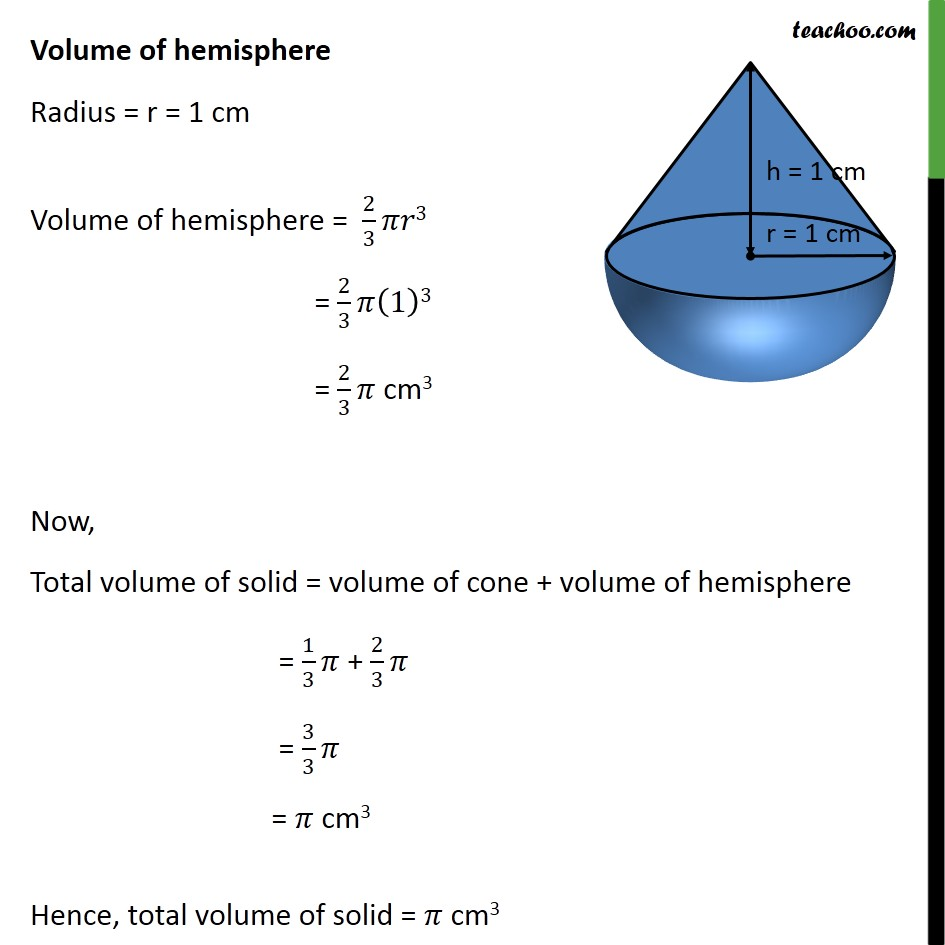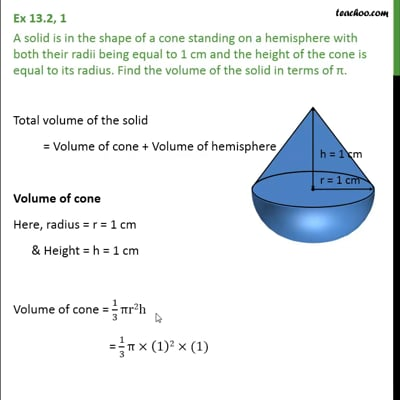Ex 13.2

Chapter 13 Class 10 Surface Areas and Volumes (Term 2)
Serial order wiseThis video is only available for Teachoo black users

### Transcript

Ex 13.2, 1 A solid is in the shape of a cone standing on a hemisphere with both their radii being equal to 1 cm and the height of the cone is equal to its radius. Find the volume of the solid in terms of . Total volume of the solid = Volume of cone + Volume of hemisphere Volume of cone Here, radius = r = 1 cm & Height = h = 1 cm Volume of cone = 1/3 r2h = 1/3 (1)2 (1) = 1/3 cm3 Volume of hemisphere Radius = r = 1 cm Volume of hemisphere = 2/3 3 = 2/3 (1)3 = 2/3 cm3 Now, Total volume of solid = volume of cone + volume of hemisphere = 1/3 + 2/3 = 3/3 = cm3 Hence, total volume of solid = cm3